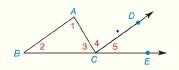Chapter 2.CT, Problem 14CTElementary Geometry For College St...

7th Edition
Alexander + 2 others
ISBN: 9781337614085

Solutions

Chapter
SectionElementary Geometry For College St...

7th Edition
Alexander + 2 others
ISBN: 9781337614085
Textbook Problem

In the figure shown, suppose that ray CD bisects exterior angle ∠ A C E of Δ A B C . If m ∠ 1 = 70 ° and m ∠ 2 = 30 ° find m ∠ 4 .To determine

To find:

The value of m4.

Explanation

According to the exterior angle property, the sum of interior angle will be equal to the sum of exterior angle.

Given:

The following figure shows the given diagram.

Figure (1)

Given that, the ray CD bisects exterior angle ACE of ΔABC. If m1=70° and m2=30° find m4.

Calculation:

The sum of interior angle will be equal to the sum of exterior angle as follows.

m1+m2=m4+m5(1)

Given that, the ray CD bisects exterior angle ACE of ΔABC

Still sussing out bartleby?

Check out a sample textbook solution.

See a sample solution

The Solution to Your Study Problems

Bartleby provides explanations to thousands of textbook problems written by our experts, many with advanced degrees!

Get Started

Find more solutions based on key concepts